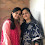Latest Articles

# Vedic Maths - The First by the First and the Last by the Last

Info Post
After a big gap, here I am again with Vedic Maths instructions. The "First by the First and the Last by the Last" is one of the sub-sutra that we will be seeing today.

Taking two simpler examples,

26 x 86 ( Condition 1: Note that the last terms are equal and the first terms add up to 10)
54 x 56 ( Condition 2: Note that the first terms are equal and the last terms add up to 10 )

According to Vedic principles, the above products falls under "First by the First and the Last by the Last" Vedic formula.

So, to solve them we have to check if any one of the conditions 1 or conditions 2 is satisfied and then proceed as follows,

Condition 1:  The last terms are equal and the first terms add up to 10
Step 1: Multiply the digits in the tens place from each number and add them to the unit digit to get your L.H.S.
Step 2: Multiply the unit digit from each number to get your R.H.S.
Step 3: Join Step 1 (L.H.S) and Step 2 (R.H.S) to get your answer.

Another example,
77 x 37 = ?
= ( 7 x 3) + 7 | 7 x 7
= 28 | 49
Therefore, 77 x 37 = 2849

Condition 2: The first terms are equal and the last terms add up to 10

Step 1: Multiply the common number (here it is 5) with that of its next number to get the L.H.S.
Step 2: Multiply the unit digits from each number to get your R.H.S.
Step 3: Join Step 1 (L.H.S) and Step 2 (R.H.S) to get your answer.

Another example,
26 x 24 = ?
= ( 2 x 3) | 6 x 4
= 6 | 24
Therefore, 26 x 24 = 624.

The above are some simple examples of the sutra. Next week, I will see you with more examples from Vedic Maths.

Share:

1.